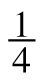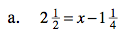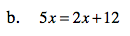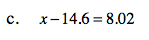### Home > CC2 > Chapter 8 > Lesson 8.1.2 > Problem8-28

8-28.
1. For each equation below, solve for x. Show the process you use to check your answer. Homework Help ✎

1. 2= x − 12. 5x = 2x + 12

3. x − 14.6 = 8.02Isolate the variable, x, and when adding, add the whole numbers as integers, but remember to convert the fractions to ones with common denominators.

$3\frac{3}{4}$Isolate the variable x.See part (b).